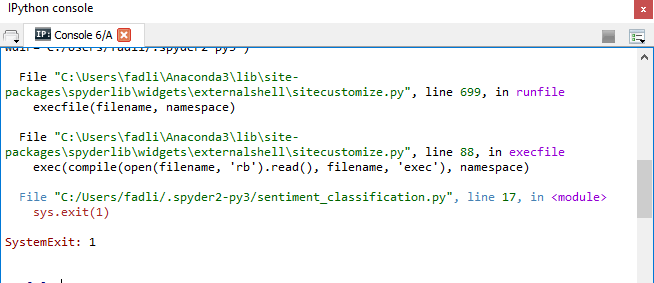{{ message }}

Instantly share code, notes, and snippets.

# bonzanini/sentiment_classification.py

Last active Oct 30, 2020
Sentiment analysis with scikit-learn
 # You need to install scikit-learn: # sudo pip install scikit-learn # # Dataset: Polarity dataset v2.0 # http://www.cs.cornell.edu/people/pabo/movie-review-data/ # # Full discussion: # https://marcobonzanini.wordpress.com/2015/01/19/sentiment-analysis-with-python-and-scikit-learn import sys import os import time from sklearn.feature_extraction.text import TfidfVectorizer from sklearn import svm from sklearn.metrics import classification_report def usage(): print("Usage:") print("python %s " % sys.argv) if __name__ == '__main__': if len(sys.argv) < 2: usage() sys.exit(1) data_dir = sys.argv classes = ['pos', 'neg'] # Read the data train_data = [] train_labels = [] test_data = [] test_labels = [] for curr_class in classes: dirname = os.path.join(data_dir, curr_class) for fname in os.listdir(dirname): with open(os.path.join(dirname, fname), 'r') as f: content = f.read() if fname.startswith('cv9'): test_data.append(content) test_labels.append(curr_class) else: train_data.append(content) train_labels.append(curr_class) # Create feature vectors vectorizer = TfidfVectorizer(min_df=5, max_df = 0.8, sublinear_tf=True, use_idf=True) train_vectors = vectorizer.fit_transform(train_data) test_vectors = vectorizer.transform(test_data) # Perform classification with SVM, kernel=rbf classifier_rbf = svm.SVC() t0 = time.time() classifier_rbf.fit(train_vectors, train_labels) t1 = time.time() prediction_rbf = classifier_rbf.predict(test_vectors) t2 = time.time() time_rbf_train = t1-t0 time_rbf_predict = t2-t1 # Perform classification with SVM, kernel=linear classifier_linear = svm.SVC(kernel='linear') t0 = time.time() classifier_linear.fit(train_vectors, train_labels) t1 = time.time() prediction_linear = classifier_linear.predict(test_vectors) t2 = time.time() time_linear_train = t1-t0 time_linear_predict = t2-t1 # Perform classification with SVM, kernel=linear classifier_liblinear = svm.LinearSVC() t0 = time.time() classifier_liblinear.fit(train_vectors, train_labels) t1 = time.time() prediction_liblinear = classifier_liblinear.predict(test_vectors) t2 = time.time() time_liblinear_train = t1-t0 time_liblinear_predict = t2-t1 # Print results in a nice table print("Results for SVC(kernel=rbf)") print("Training time: %fs; Prediction time: %fs" % (time_rbf_train, time_rbf_predict)) print(classification_report(test_labels, prediction_rbf)) print("Results for SVC(kernel=linear)") print("Training time: %fs; Prediction time: %fs" % (time_linear_train, time_linear_predict)) print(classification_report(test_labels, prediction_linear)) print("Results for LinearSVC()") print("Training time: %fs; Prediction time: %fs" % (time_liblinear_train, time_liblinear_predict)) print(classification_report(test_labels, prediction_liblinear))

###mmuppidi commented Nov 2, 2015

 Hello Marco Bonzanini, Thanks for the tutorial, I got this error when I was going through the tutorial. What do you think has caused it ? Is it because of the data set or may be some thing has changed in Scikit learn since your tutorial has been posted ? Please let me know. ``````Traceback (most recent call last): File "sentiment_classification.py", line 54, in train_vectors = vectorizer.fit_transform(train_data) File "/Library/Python/2.7/site-packages/sklearn/feature_extraction/text.py", line 1285, in fit_transform X = super(TfidfVectorizer, self).fit_transform(raw_documents) File "/Library/Python/2.7/site-packages/sklearn/feature_extraction/text.py", line 804, in fit_transform self.fixed_vocabulary_) File "/Library/Python/2.7/site-packages/sklearn/feature_extraction/text.py", line 739, in _count_vocab for feature in analyze(doc): File "/Library/Python/2.7/site-packages/sklearn/feature_extraction/text.py", line 236, in tokenize(preprocess(self.decode(doc))), stop_words) File "/Library/Python/2.7/site-packages/sklearn/feature_extraction/text.py", line 113, in decode doc = doc.decode(self.encoding, self.decode_error) File "/System/Library/Frameworks/Python.framework/Versions/2.7/lib/python2.7/encodings/utf_8.py", line 16, in decode return codecs.utf_8_decode(input, errors, True) UnicodeDecodeError: 'utf8' codec can't decode byte 0xe8 in position 79: invalid continuation byte ``````

###mmuppidi commented Nov 2, 2015

 From the SciKit learn docs I have learnt that if byte sequence provided to analyze, contains characters from different encoding then it will raise 'UnicodeDecodeError'. The simplest way of avoiding this is by using `decode_error='ignore'` parameter. So replacing line 50 with below line would fix the problem. ``````vectorizer = TfidfVectorizer(min_df=5, max_df = 0.8, sublinear_tf=True, use_idf=True,decode_error='ignore') `````` Thanks once again. Its a nice tutorial for beginners.

###bonzanini commented Nov 9, 2015

 @mk01github The code was developed and tested on Python 3 rather than 2.7, that's often a source of encoding problems

###FrendyAr commented Aug 30, 2017why i got this error ? help me please.... thanks